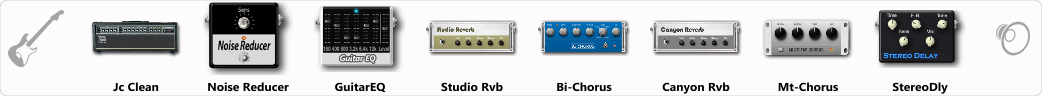# Guitar lite

Discussion in 'ToneLib-GFX presets' started by Mrc, Jun 23, 2022.

1. Guitar lite

Preset name: Empty Preset

Effects chain:Effect: "Jc Clean" (Amp simulators), active - "yes"
"Gain" = 50
"Bass" = 50
"Middle" = 50
"Treble" = 50
"Presence" = 50
"Master" = 50
"Output" = 50
"Level (dB)" = 0

Effect: "Noise Reducer" (Dynamics / Filter), active - "yes"
"Sens" = 100
"Mode" = Hard

Effect: "GuitarEQ" (Dynamics / Filter), active - "yes"
"160 Hz" = 0
"400 Hz" = 0
"800 Hz" = 0
"1.6 kHz" = 0
"3.2 kHz" = 0
"6.4 kHz" = 0
"12 kHz" = 0
"Level (dB)" = 0

Effect: "Studio Rvb" (Reverb), active - "yes"
"Time" = 9.3
"PreDelay" = 32
"LoDamp" = 0
"HiDamp" = 12
"Mix" = 58

Effect: "Bi-Chorus" (Modulation / Sfx), active - "yes"
"Speed A" = 3.2
"Speed B" = 4.0
"Depth" = 43
"Reso" = 0
"Mode" = Parallel
"Mix" = 56

Effect: "Canyon Rvb" (Reverb), active - "yes"
"Time" = 7.3
"PreDelay" = 42
"LoDamp" = 0
"HiDamp" = 32
"Mix" = 39

Effect: "Mt-Chorus" (Modulation / Sfx), active - "yes"
"Speed" = 5.3
"Depth" = 92
"Time" = 2.5
"Mix" = 67

Effect: "StereoDly" (Delay), active - "yes"
"Time" = 397
"Feedback" = 63
"Tone" = 100
"Sens" = 0
"Mix" = 68

Note: You will need to download and install the ToneLib-GFX software to use the preset.

#### Attached Files:

• ###### Guitar_lite.tlgfx
File size:
689 bytes
Views:
1,906
vrmlnxt likes this.